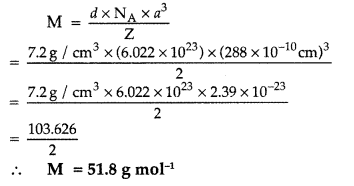# Solid State Chemistry PDF Notes

## Solid State Chemistry PDF Notes

Solid State Chemistry Class 12 Important Questions with Answers free pdf solid state questions pdf solid state questions and answers solid state questions for neet pdf solid state question bank with answers solid state previous year questions solid state questions jee solid state pdf solid state class 12 types of solid state solid state ppt solid state imp mcqs solid state physics solid state questions for neet solid state questions for neet pdf download solid state questions for neet pdf solid state questions pdf solid state questions and answers pdf solid state questions for jee mains solid state questions iit jee pdf solid state questions iit jee solid state questions mcq jee mains solid state questions plus two chemistry solid state questions csir net solid state questions class 12 solid state questions jee advanced solid state questions cbse solid state questions neet 2019 solid state questions ncert solid state questions

#### The Solid State Class 12 Important Questions Very Short Answer Type

Question 1.
Which point defect in crystals does not alter the density of the relevant solid?
Frenkel defect.

Question 2.
Which point defect in its crystal units alters the density of a solid?

Schottky defect.

Question 3.
Which point defect in its crystal units increases the density of a solid?
Metal excess defect increases the density of a solid. It is due to presence of extra cations in the interstitial sites.

Question 4.
How do metallic and ionic substances differ in conducting electricity?

The electrical conductivity in metallic substances is due to free electrons while in ionic substances the conductivity is due to presence of ions.

Question 5.
Which point defect of its crystals decreases the density of a solid?
Schottky defect.

Question 6.
What is the number of atoms in a unit cell of a face-centred cubic crystal?

The number of atoms in a unit cell of fcc-crysta! is 4 atoms.

Question 7.
Write a feature which will distinguish a metallic solid from an ionic solid.

The electrical conductivity in metallic solid is due to free electrons while in ionic solid the conductivity is due to presence of ions.

Question 8.
Which point defect in crystals of a solid does not change the density of the solid?

Frenkel defect.

Question 9.
Which point defect in crystals of a solid decreases the density of the solid?

Schottky defect.

Question 10.
What type of interactions hold the molecules together in a polar molecular solid?

Dipole-dipole forces of attractions hold the molecules together in a polar molecular solid.

Question 11.
What type of semiconductor is obtained when silicon is doped with arsenic?

n-type semiconductor.

Question 12.
Write a distinguishing feature of metallic solids.

Metallic solids possess high electrical and thermal conductivity due to presence of free electrons.

Question 13.
‘Crystalline solids are anisotropic in nature.’ What does this statement mean?

It means that crystalline solids show different values of their some properties like electrical conductivity, refractive index, thermal expansion etc. in different directions.

Question 14.
Which stoichiometric defect in crystals increases the density of a solid?

Interstitial defect in crystals increases the density of a solid.

Question 15.
What is meant by ‘doping’ in a semiconductor?

Addition of a suitable impurity to a semiconductor to increase its conductivity is called doping.

Question 16.
Write a point of distinction between a metallic solid and an ionic solid other than metallic lustre.
Metallic solid conducts electricity in solid state but ionic solids do so only in molten state or in solution or metals conduct electricity through electrons and ionic substances through ions. Metallic solids are malleable and ductile while ionic solids are hard and brittle.

Question 17.
How may the conductivity of an intrinsic semiconductor be increased?

The conductivity is increased by adding an appropriate amount of suitable impurity. This process is called as intrinsic doping.

Question 18.
Which stoichiometric defect increases the density of a solid?
Interstitial defect increases the density of a solid.

Question 19.
What are n-type semiconductors?

n-type semiconductor : They are obtained by doping silicon with an element of group15, like P, As etc.

Question 20.
What type of stoichmetric defect is shown by AgBr and Agl ?

AgBr shows both Frenkel defect and Schottky defect whereas Agl shows Frenkel defect.

Question 21.
What type of defect can arise when a solid is heated ?

Vacancy defects can arise when a solid is heated.

Question 22.
Why does LiCl acquire pink colour when heated in Li vapours?

This is due to metal excess defect due to anionic vacancies in which the anionic sites are occupied by unpaired electrons (F-centres).

Question 23.
How many atoms constitute one unit cell of a face-centered cubic crystal?

4 atoms constitute one unit cell of a fee crystal.

Question 24.
What type of stoichiometric defect is shown by AgCl?

Frenkel defect is shown by AgCl.

Question 25.
What type of substances would make better Permanent Magnets: Ferromagnetic or Ferrimagnetic?

Ferromagnetic substances would make better I permanent magnets
Example : Fe, Co, Ni etc.

Question 26.
Calculate the number of atoms in a face centred cubic unit cell.

In face centered cubic arrangement, number of lattice points are : 8 + 6.
∴ Lattice points per unit cell = 8×18+6×12 = 4

Question 27.
On heating a crystal of KC1 in potassium vapour, the crystal starts exhibiting a violet colour. What is this due to?

The Cl ions diffuse to the surface and combine j with atoms which get ionized by losing electrons. ! These electrons are trapped in anions vacancies j and act as F-centre which imparts violet colour to the crystal.

Question 28.
Which type of ionic substances show Schottky defect in solids?

Highly ionic compounds with high coordination rjuniber and small difference in size of cations and anions show schottky defect.

Question 29.
How many atoms per unit cell (z) are present in bcc unit cell?

Number of atoms in a unit cell of a body centred cubic structure :
Contribution by 8 atoms on the corners
= 18 × 8 = 1
Contribution by the atom presents within the body = 1
∴ Total number of atoms present in the unit cell = 1 + 1 = 2 atoms

Question 30.
What type of stoichiometric defect is shown by NaCl?

Schottky defect is shown by NaCl.

Question 31.
Write a distinguishing feature between a metallic solid and an ionic solid.

The electrical conductivity in metallic substances is due to free electrons while in ionic substances the conductivity is due to presence of ions.

Question 32.
Why are crystalline solids anisotropic?

Crystalline solids show different values of their some properties like electrical conductivity, refractive index, thermal expansion etc. in different directions.

Question 33.
What is meant by ‘antiferromagnetism’?

Antiferromagnetism : These substances possess zero net magnetic moment because of presence of equal number of electrons with opposite spins.

Question 34.
Write a distinguishing feature of a metallic solid compared to an ionic solid.

Metallic solid conducts electricity in solid state but ionic solids do so only in molten state or in solution or metals conduct electricity through electrons and ionic substances through ions. Metallic solids are malleable and ductile while ionic solids are hard and brittle.

Question 35.
What is the formula of a compound in which the element Y forms ccp lattice and atoms of X occupy 1/3rd of tetrahedral voids?

Formula is X2Y3.

Question 36.
What is the formula of a compound in which the element Y forms ccp lattice and atoms of X occupy 2/3rd of tetrahedral voids?

Y atoms are N (No. of tetrahedral voids are 2N), No. of tetrahedral voids occupied by X are
23 × 2N = 4N3
X : Y = 4N : 3N
Formula : X4Y3

Question 37.
What is the no. of atoms per unit cell (z) in a body-centred cubic structure?

Contribution by the atoms present at eight comers = 8 × 18 = 1
Contribution by the atoms present at centre = 1
Total number of atoms present in unit cell = 1 + 1 = 2

Question 38.
What type of stoichiometric defect is shown by AgCl?

AgCl shows Frenkel defect.

Question 39.
What type of magnetism is shown by a substance if magnetic moments of domains are arranged in same direction?

Ferromagnetism is shown by a substance if magnetic moments of domains are arranged in same direction.Question 40.
Give an example each of a molecular solid and an ionic solid.

Molecular solid → Iodine (I2)
Ionic solid → Sodium chloride (NaCl)

Question 41.
A metallic element crystallises into a lattice having a pattern of AB AB … and packing of spheres leaves out voids in the lattice. What type of structure is formed by this arrangement?

Tetrahedral void is formed in AB AB … pattern. The hexagonal close packing (hep) is formed in this arrangement.

Question 42.
A metallic element crystallises into a lattice having a ABC ABC … pattern and packing of spheres leaves out voids in the lattice. What type of structure is formed by this arrangement?

Octahedral voids are formed in ABC ABC … pattern. The cubic close packing (ccp) is formed in this arrangement.

Question 43.
What type of Stoichiometric defect is shown by AgCl?
Frenkel defect.

Question 44.
What type of stoichiometric defect is shown by NaCl?

Schottky defect is shown by NaCl.

Question 45.
Which ionic compound shows both Frenkel and Schottky defects?

Silver bromide (AgBr) shows both Schottky and Frenkel defect.

#### The Solid State Class 12 Important Questions Short Answer Type – I (SA – 1)

Question 46.
Explain how you can determine the atomic mass of an unknown metal if you know its mass density and the dimensions of unit cell of its crystal.

Suppose edge of the unit cell = a pm
Number of atoms present per unit cell = Z
∴ Volume of unit cell = (a pm)3
= (a × 10-10cm)3 = a3 × 10-30 cm3
Density of unit cell =  Mass of unit cell  Volume of unit cell  ……………… (i)
Mass of unit cell = Number of atoms in the unit cell × mass of each atom
= Z × m
Mass of each atom =  Atomic mass  Avogadro’s no. =MN0
Substituting these values in equation (i), we get
Density of unit cell = Z×Ma3×10−30×N0
If a is in cm, d = Z×Ma3×N0 g/cm3
∴ Molar mass can be calculated as
M = d×a3×N0Z

Question 47.
Calculate the packing efficiency of a metal crystal for a simple cubic lattice.

Percentage efficiency of packing of simple cubic lattice = 52.4%.

Question 48.
Define the following terms in relation to crystalline solids :
(i) Unit cell (ii) Coordination number Give one example in each case. (All India) 2011
(i) Unit cell : The smallest three dimensional portion of a complete space lattice which when repeated over and again in different directions produces the complete space lattice is called the unit cell.
Example: Cubic unit cell, Hexagonal unit cell etc.
(ii) Coordination number : The number of nearest spheres with which a particular sphere is in contact is called co-ordination number.
Example : Co-ordination number of hexagonal (hep) structures is 12.

Question 49.
The unit cell of an element of atomic mass 108 u and density 10.5 g cm-3 is a cube with edge length, 409 pm. Find the type of unit cell of the crystal. [Given : Avogadro’s constant = 6.023 × 1023 mol-1]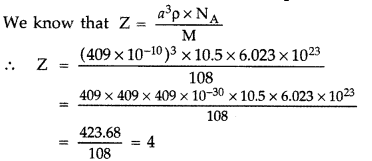So it forms cubic- closed packed (ccp) lattice or fee structure.

Question 50.
Explain the following terms with suitable examples : Ferromagnetism and Ferrimagnetism

Ferromagnetic solids : The solids which are strongly attracted by external magnetic field and do not lose their magnetism when the external field is removed are called ferromagnetic solids. The property, thus exhibited, is termed as ferromagnetism.
Example: Fe, Co and Ni show ferromagnetism at room temperature.

Ferrimagnetic solids : The solids which are expected to show large magnetism due to the presence of unpaired electrons but in fact have small net magnetic moment, are called ferrimagnetic solids.
Example : Fe3O4 and ferrites.

Question 51.
An element X crystallizes in f.c.c structure. 208 g of it has 4.2832 × 1024 atoms. Calculate the edge of the unit cell, if density of X is 7.2 g cm-3.

Z = 4(fcc) A = 7,2 g/cm3 a = ?
4.2832 × 1024 atoms have mass = 208 g
6.022 × 1023 atoms have mass
= 2084.2832×1024 × 6.022 × 1023 = 29.24 (at. mass)
a3 = Z×Md×NA=4×29.247.2×6.022×1023
= 269.6 x 10-24 cm3
∴ a = 6.46 x 10-8 cm = 6.46 Å

Question 52.
What is a semiconductor? Describe the two main types of semiconductors.

Semiconductor : The solid materials whose electrical conductivity lies between those of the typical metallic conductors and insulators are termed as semiconductors. The semiconductors possess conductivity in the range of 102 to 10-9 ohm-1 cm-1.
These are of two types :
(a) n-type semiconductors : Doping of higher group element impurity forms n-type semiconductors, e.g. when ‘As’ is doped in ‘Ge’.
(b) p-type semiconductors : Impurity of lower groups forms electron deficient bond in the structure. Electron deficiency develops to p-hole.

Question 53.
Account for the following:
(i) Schottky defects lower the density of related solids.
(ii) Conductivity of silicon increases on doping it with phosphorus.

(i) Schottky defect produced due to missing of equal number of cation and anion from lattice as a result of which the density of the lattice solid decreases.
(ii) The conductivity of silicon increases due to negatively charged extra electron of doped pentavalent phosphorus.

Question 54.
Aluminium crystallizes in an fee structure. Atomic radius of the metal is 125 pm. What is the length of the side of the unit cell of the metal?

For fee, Formula : r = a22√
Given: r = 125 pm
∴ a = 22–√r ÷ a = 22–√ × 125
⇒ a = 2 × 1.414 × 125 = 353.5 pm

Question 55.
(a) Why does presence of excess of lithium makes LiCl crystals pink?

(b) A solid with cubic crystal is made of two elements P and Q. Atoms of Q are at the corners of the cube and P at the body-centre. What is the formula of the compound? (All India) 2013
(a) This is due to metal excess defect due to anionic vacancies in which the anionic sites are occupied by unpaired electrons (F-centres).
(b) As atoms of Q are present at the 8 centres of the cube, therefore, number of atoms of Q in the unit cell = 18 × 8 = 1
The atom P is at the body centre .-. Number of atoms = 1
Ratio of atoms P : Q = 1 : 1
Hence, the formula of the compound is PQ.

Question 56.
(a) What change occurs when AgCl is doped with CdCl2?

(b) What type of semiconductor is produced when silicon is doped with boron? (All India) 2013
(a) Impurity defect of ionic solids is produced when AgCl is doped with CdCl2. Due to this defect vacancies are created that result in higher electrical conductivity of the solid.
(b) p-type semi-conductor is obtained when silicon is doped with boron.

Question 57.
If NaCl is doped with 10-3 mole percent SrCl2, what will be the concentration of cation vacancies? (NA = 6.02 × 1023 mol-1)
10-3 mol percent means 100 moles of NaCl are doped with 10-3 moles of SrCl2
∴ 1 mole of NaCl is doped with SrCl2
= 10−3100 = 10-5 mole
Since each Sr2+ ion introduces one cation vacancy
∴ Concentration of cation vacancies
= 10-5 mol/mol of NaCl
= 10-5 × 6.02 × 1023 mol-1
= 6.02 × 1018 mol-1

Question 58.
What is a semiconductor? Describe the tivo main types of semiconductors and contrast their conduction mechanism.
Semiconductor : The solids which have intermediate conductivities between metals and non-metals i,e. between 10-6 to 104 π-1 m-31 are called semiconductors.
Example : Germanium and Silicon.
Main types of semiconductors are of two types :
(i) Intrinsic semiconductor : These are insulators at room temperature and become semiconductors when temperature is raised
(ii) Extrinsic semiconductor :
p-type semiconductor
n-type semiconductor
These are formed by dropping impurity of lower or higher group.
These are subdivided into two types :
• p-type semiconductor : When a silicon crystal is doped with atoms of group-13 elements like B, Al, Ga etc., the atom forms only 3 covalent bonds with the Si atom and 4th missing electron creates a hole which conducts electricity.
• n-type semiconductor : When a silicon crystal is doped with atoms of group-15 elements like P, As etc., then only four of the five valence electrons of each impurity atom, participate in 4 covalent bond formation and 5th e conducts electricity.

Question 59.
A compound forms hep structure. What is the total number of voids in 0.5 mol of it? How many of these are tetrahedral voids?

No. of atoms in the hep = 0.5 × 6.022 × 1023
= 3.011 × 1023
No. of octahedral voids
= No. of atoms in packing = 3.011 × 1023
No. of tetrahedral voids
= 2 × No. of atoms in packing
= 2 × 3.011 × 1023 = 6.022 × 1023
∴ Total no. of voids
= 3.011 × 1023 + 6.022 × 1023 = 9.033 × 1023

Question 60.
An element crystallizes in a structure having fee unit cell of an edge 200 pm. Calculate the density if 200 g of this element contains 24 × 1023 atoms.

24 × 1023 atoms of an element have mass = 200 g
∴ 6.022 × 1023 atoms of an element have mass
= 20024×1023 × 6.022 × 1023 = 50.18 g
Given : a = 200 pm = 200 × 10-12 cm,
Z = 4 (For fee), M = 50.18 g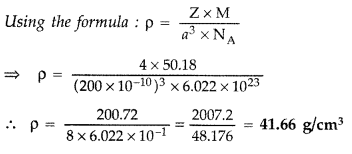Question 61.
An element with density 11.2 g cm-3 forms a f.c.c. lattice with edge length of 4 × 10-8 cm. Calculate the atomic mass of the element. (Given : NA = 6.022 × 1023 mol-1)

Given : p = 11.2 g cm-3, a = 4 × 10-8 cm
For fee lattice, Z = 4
Using formula,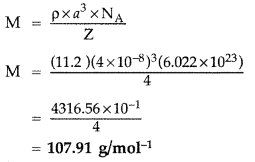Question 62.
Examine the given defective crystal 2014Answer the following questions :
(i) What type of stoichiometric defect is shown by the crystal?
(ii) How is the density of the crystal affected by this defect?
(tii) What type of ionic substances show such defect? (Delhi)
(i) Schottky defect
(ii) Density of the crystal decreases
(iii) NaCl (Ionic solids having approximate equal size of cations and anions)

Question 63.
An element with density 2.8 g cm-3 forms a f.c.c. unit cell with edge length 4 × 10-8 cm. Calculate the molar mass of the element.
(Given : NA = 6.022 × 1023mol-1) (All India) 2014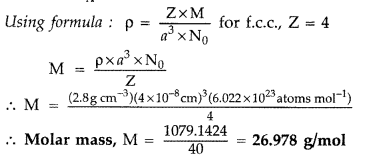Question 64.
(i) What type of non-stoichiometric point defect is responsible for the pink colour of LiCl?
(ii) What type of stoichiometric defect is shown by NaCl?

(i) This is due to metal excess defect due to anionic vacancies in which the anionic sites are occupied by unpaired electrons (F-centres).
(ii) Schottky defect is shown by NaCl.

Question 65.
How will you distinguish between the following pairs of terms :
(i) Tetrahedral and octahedral voids
(ii) Crystal lattice and unit cell.

(ii) A regular arrangement of the constituent particles of a crystal in a three dimensional space is called crystal lattice.
The smallest three dimensional portion of a complete crystal lattice, which when repeated over and again in different directions produces the complete crystal lattice is called the unit cell.

Question 66.
(i) Write the type of magnetism observed when the magnetic moments are appositively aligned and cancel out each other.
(ii) Which stoichiometric defect does not change » the density of the crystal?

1. Diamagnetism is observed when the magnetic moments are oppositively aligned and cancel out each other.
2. Frenkel defect does not change the density of the crystal.

Question 67.
(i) Write the type of magnetism observed when the magnetic moments are aligned in parallel and anti-parallel directions in unequal numbers.
(ii) Which stoichiometric defect decreases the density of the crystal?

1. Ferrimagnetism is observed.
2. Schottky defect decreases the density of the crystal.

68. Define the following terms:
(i) n-type semiconductor
(ii) Ferrimagnetism
(i) n-type semiconductor : When Si/Ge is doped with group 15 element.
(ii) Ferrimagnetism : When magnetic domains are aligned in parallel and anti-parallel directions in unequal numbers.

Question 69.
Explain the following terms with suitable examples :
(i) Frenkel defect (ii) F-centres

(i) Frenkel defect : The defect in which the smaller ion/cation is dislocated to a nearby interstitial site.
Example : Silver halides, ZnS.
(ii) F-centres : The anion vacancy occupied by an electron is called F-centre in Alkali metal halides.
Example : NaCl, KC1, Li Cl.

Question 70.
Calculate the number of unit cells in 8.1 g of aluminium if it crystallizes in a face-centered cubic (f.c.c.) structure. (Atomic mass of Al = 27 g mol-1)

1 mole of Aluminium = 27 g = 6.022 × 1023
Hence, No. of atoms present in 27 g of Al
= 6.022×102327
As f.c.c. unit cell contains 4 atoms
∴ No. of f.c.c. unit cells present
= 6.022×1023×8.127×4
= 0.45165 × 1023 = 4.5165 × 1022

Question 71.
Iron has a body centred cubic unit cell with a cell edge of 286.65 pm. The density of iron is 7.87 g cm-3. Use this information to calculate Avogadro’s number (At. mass of Fe = 56 g mol-1).

Given :
a = 286.65 pm = 286.65 × 10-10,
d = 7.87 g cm-3, M = 56 g mol-1
Z = 2 NA = ?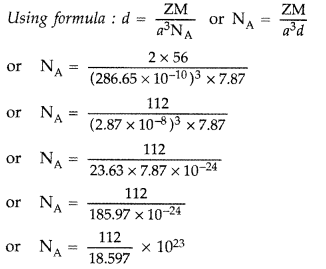∴ Avogadro’s number NA = 6.022 × 1023

Question 72.
Silver crystallises with face-centred cubic unit cells. Each side of the unit cell has a length of 409 pm. What is the radius of an atom of silver? (Assume that each face atom is touching the four comer atoms.)

Given : a = 409 pm r = ?
For fee unit cell, the formula is a
r = a22√
or r = 40922√=4092×1.414=4092.828
∴ r = 144.62
∴ Radius of an atom of silver = 144.62 pm

Question 73.
The well known mineral fluorite is chemically calcium fluoride. It is known that in one unit cell of this mineral there are
4 Ca2+ ions and 8 F ions and that Ca2+ ions are arranged in a fee lattice. The F ions fill all the tetrahedral holes in the face centred cubic lattice of Ca2+ ions. The edge of the unit cell is 5.46 × 10-8 cm in length. The density of the solid is 3.18 g cm-3. Use this information to calculate Avogadro’s number (Molar mass of CaF2 = 78.08 g mol-1).
Given
Edge of the unit cell (a) = 5.46 × 10-8 cm
Density (P) = 3.18 g cm-3
According to the formula ;Question 74.
The density of copper metal is 8.95 g cm-3. If the radius of copper atom is 127.8 pm, is the copper unit cell a simple cubic, a body-centred cubic or a face centred cubic structure?

(Given : At. mass of Cu = 63.54 g mol-1 and NA = 6.02 × 1023 mol-1)
If copper atom were simple cubic :
a = 2 × r = 2 × 127.8 pm = 255.6 pm
= 255.6 pm = 255.6 × 10-10 cm
Z = 1
p = Z×Ma3×NA=1×63.54(255.6×10−10)3×(6.02×1023)
∴ P = 6.34 g cm-3
Actual density = 8.95 g cm-3
Hence copper atom is not simple cubic.
If copper atom were body-centred :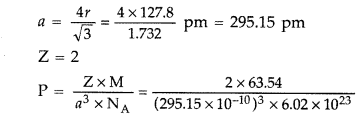∴ P = 8.21 g cm-3
Hence, copper atom is not body centered
If copper atom were face-centered
a = 22–√
or a = 2 × 1.414 × 127.8 pm
= 361.4 pm = 361.4 × 10-10 cm
P = Z×Ma3×NA=4×63.54(361.4×10−10)3×6.02×1023
∴ P = 8.94 cm-3
Hence, copper is face-centred cubic.

Question 75.
Silver crystallises in face-centred cubic unit cells. Each side of the unit cell has a length of 409 pm. What is the radius of silver atom?

Refer to Q. 72, Page 9
Given : a = 409 pm r = ?
For fee unit cell, the formula is a
r = a22√
or r = 40922√=4092×1.414=4092.828
∴ r = 144.62
∴ Radius of an atom of silver = 144.62 pm

Question 76.
Silver crystallizes in face-centered cubic unit cell. Each side of this unit cell has a length of 400 pm. Calculate the radius of the silver atom. (Assume the atoms just touch each other on the diagonal across the face of the unit cell. That is each face atom is touching the four comer atoms.)

Given : a = 400 pm, r = ?
For fee unit cell : r = a22√
or r = 4002×1.4142=4002.828 ∴ r = 141.4
∴ Radius of the silver, r = 141.4 pm

Question 77.
The density of lead is 11.35 g cm-3 and the metal crystallizes with fee unit cell. Estimate the radius of lead atom.
(At. Mass of lead = 207 g mol-1 and NA = 6.02 × 1023 mol-1)

Given : d = 11.35 g cm-3
According to the formulaQuestion 78.
Tungsten crystallizes in body centred cubic unit cell. If the edge of the unit cell is 316.5 pm, what is the radius of tungsten atom?
For bee, unit cell : radius, r = 3√a4 (∵ a = 316.5 pm)
= 3√×316.5pm4=1.732×316.5pm4 = 137 pm

Question 79.
Iron has a body centred cubic unit cell with a cell dimension of 286.65 pm. The density of iron is 7.874 g cm-3. Use this information to calculate Avogadro’s number. (At mass of Fe = 55.845 u)∴ Avogadro’s number, NA = 6.02 × 1023 mol-1

Question 80.
Copper crystallises with face centred cubic unit cell. If the radius of copper atom is 127.8 pm, calculate the density of copper metal.
(Atomic mass of Cu = 63.55 u and Avogadro’s number NA = 6.02 × 10223 mol-1)

In fee, lattice, Z = 4 atoms [fee = face centred cubic]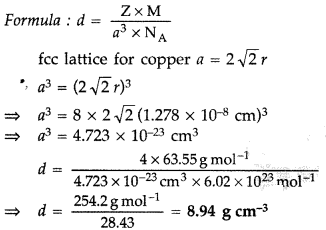Question 81.
Iron has a body centred cubic unit cell with the cell dimension of 286.65 pm. Density of iron is 7.87 g cm-3. Use this information to calculate Avogadro’s number. (Atomic mass of Fe = 56.0 u)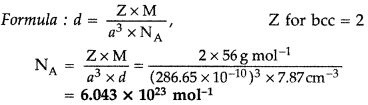Question 82.
(a) Some of the glass objects recovered from ancient monuments look milky instead of being transparent. Why?
(b) Iron (II) oxide has a cubic structure and each side of the unit cell is 5Å. If density of the oxide is 4 g cm-3, calculate the number of Fe2+ and O2- ions present in each unit cell. [Atomic mass : Fe = 56 u, O = 16 u; Avagadro’s number = 6.023 × 1023 mol-1]

(a) Some of the glass objects found from ancient monuments look to be milky in appearance because of crystallisation of glass.
(b) Volume of unit cell = a3 = (5 A) = (5 × 10-8)3
= 1.25 × 10-22 cm
Density of FeO = 4g cm-3
Mass of unit cell = Volume × Density
= 1.25 × 10-22 × 4 g
= 5 × 10-22 g
Mass of FeO molecule per unit cell
= 5×10−22g1.195×10−22g = 4.19 ≈ 4
Thus 4Fe+2, 4O-2 will be present in each unit cell.

Question 83.
(a) What are intrinsic semi-conductors? Give an example.
(b) What is the distance between Na+ and Cl ions in NaCl crystal if its density is 2.165 g cm-3? [Atomic Mass of Na = 23u, Cl = 35.5u; Avogadro’s number = 6.023 × 1023]

(a) Intrinsic semi-conductors : These are insulators at room temperature and become semi-conductors when temperature is raised, Example : silicon and germanium.
(b) Applying the formula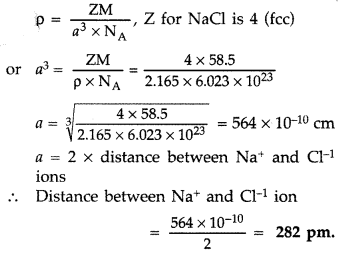Question 84.
(a) What type of semiconductor is obtained when silicon is doped with boron?
(b) What type of magnetism is shown in the following alignment of magnetic moments?(c) What type of point defect is produced when AgCl is doped with CdCl2?

(a) p-type semi-conductor is obtained when silicon is doped with boron.
(b) Ferromagnetism is shown when the alignment of magnetic movements will be(c) Impurity defect of inonic solids is produced when AgCl is doped with CdCl2. Due to this defect vacancies are created that result in higher electrical conductivity of the solid.

Question 85.
An element occurs in bcc structure. It has a cell edge length of 250 pm. Calculate the molar mass if its density is 8.0 g cm-3. Also calculate the radius of an atom of this element.

Given : For bcc structure, Z = 2
Edge of the unit cell, a = 250 pm
Density of the element, p = 8.0 g/ cm3
M = ? r = ?
Using the formulaQuestion 86.
Iron (II) oxide has a cubic structure and each unit cell has a size of 5 Å. If density of this oxide is 4 g cm-3, calculate the number of Fe2+ and O2- ions present in each unit cell.
(Atomic mass of Fe = 56, O = 16, NA = 6.023 × 1023 and 1 Å = 10-8 cm)

Given : p = 4g cm-3
a = 5Å = 5 × 10-8 cm M = 72 g/’mol, Z = ?
Using the formula for cubic crystals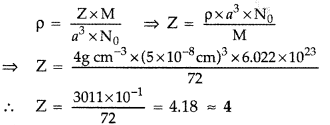There are four formula units of FeO present per unit cell. Hence it has face-centred cubic lattice where each Fe2+ and O2- are four in number.

Question 87.
Niobium crystallizes in body-centred cubic structure. If its density is 8.55 g cm-3, calculate atomic radius of niobium, given its atomic mass 93u.

Given : p = 8.55 g cm3,
M = 93 g/mol-1
Z = 2 (For bcc) a = ?
Using formula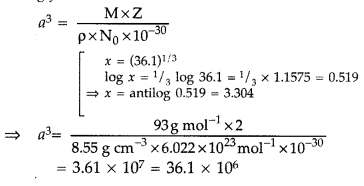a = (36.1)1/3 × 102 ρm
∴ a = 3.304 × 102 ρm = 330.4 ρm
For bcc r1 = 3√4 a = 3√4 × 330.4 = 143.1 ρm

Question 88.
The density of copper is 8.95 g cm-3. It has a face centred cubic structure. What is the radius of copper atom?
(Atomic mass Cu = 63.5 g mol-1, NA = 6.02 × 1023 mol-1)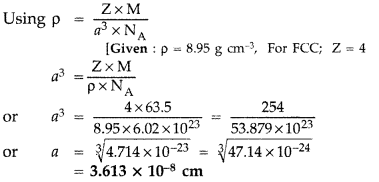Question 89.
Iron has a body centred cubic unit cell with a cell dimension of 286.65 pm. The density of iron is 7.874 g cm-3. Use this information to calculate Avogadro’s number.
(Atomic mass of Fe = 55.84 g mol-1)

Given :
a = 286.65 pm = 286.65 × 10-10,
d = 7.87 g cm-3, M = 56 g mol-1
Z = 2 NA =?∴ Avogadro’s number NA = 6.022 × 1023

Question 90.
Iron has a body centred cubic unit cell with a cell dimension of 286.65 pm. The density of iron is 7.874 g cm-3. Use this information to calculate Avogadro’s number.
(Gram atomic mass of Fe = 55.84 g mol-1).

Given :
a = 286.65 pm = 286.65 × 10-10,
d = 7.87 g cm-3, M = 56 g mol-1
Z = 2 NA = ?∴ Avogadro’s number NA = 6.022 × 1023

Question 91.
An element with molar mass 27 g mol-1 forms a cubic unit cell with edge length 4.05 × 10-8 cm. If its density is 2.7 g cm-3, what is the nature of the cubic unit cell?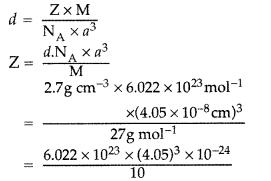= 6.022 × 10-2 × 66.43 = 4.0004 = 4
It has Face centred cubic cell/fee.

Question 92.
Examine the given defective crystal :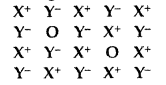Answer the following questions :
(i) Is the above defect stoichiometric or non- stoichiometric?
(ii) Write the term used for this type of defect. Give an example of the compound which shows this type of defect.
(iii) How does this defect affect the density of the crystal?

(i) It is stoichiometric defect.
(ii) Schottky defect, e.g. NaCl.
(iii) Density of crystal decreases.

Question 93.
Define the following :
(i) Schottky defect
(ii) Frenkel defect
(iii) F-centre

(i) Schottky defect: If in an ionic crystal of type A B , equal number of cations and anions are missing from their lattice sites so that the electrical neutrality is maintained, it is called Schottky defect.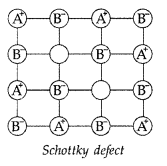(ii) Frenkel defect : If an ion leaves its site from its lattice site and occupies the interstitial site and maintains electrical neutrality, then it is called Frenkel defect.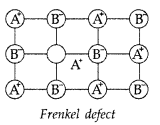(iii) F-centre : The centres which are created by trapping of electrons in anionic vacancies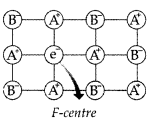and which are responsible for imparting colour to the crystals are called F-centres. (F = Fabre)

Question 94.
Silver crystallises in fee lattice. If edge length of the unit cell is 4.077 × 10-8 cm, then calculate the radius of silver atom.

Given : a = 4.077 × 10-8 cm r = ? for fee lattice
Using formula,
Radius (r) = a22√
r = 4.077×10−82×1.414cm=4.077×10−82.828
∴ r = 1.441 × 10-8 cm

Question 95.
An element crystallizes in a f.c.c. lattice with cell edge of 250 pm. Calculate the density if 300 g of this element contains 2 × 1024 atoms.

Given: a = 250 pm = 250 × 10-10 cm
z = 4 (for fee)
M = ? d = ?
Using formula : d = z×Ma3NA
∵ 2 × 1024 atoms of an element have mass = 300g
∴ 6.022 × 1023 atoms of an element have mass
= 300×6.022×10232×1024 = 90.33 g
Now M = 90.33 g

Question 96.
An element crystallizes in a b.c.c. lattice with cell edge of 500pm. The density of the element is 7.5g cm-3. How many atoms are present in 300 g of the element?

Given: For b.c.c. structure, z = 2
Edge of the unit cell, a = 500 pm = 500 × 1010 cm
Density d = 7.5 g cm-3
Using the formula,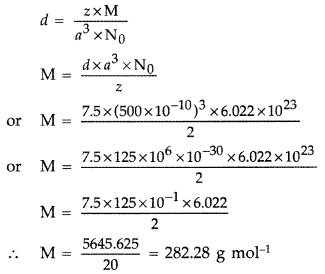∵ 282.28 g of the element contains = 6.022 × 1023 atoms
∴ 300 g of the element contains
= 6.022×1023282.28 × 300
= 1806.6282.28×1023 × 1023 = 6.40 × 1023 atoms

Question 97.
If NaCl is doped with 10-3 mol % of SrCl2, what is the concentration of cation vacancies?

Concentration of SrCl2 = 10-3 mol% = 10-3/100 mol = 10-5 mol
1 mol of NaCl on doping procuces = 6.022 × 1023 cation vacancies
Therefore, 10-5 mol of NaCl on doping produces = 6.022 × 1023 × 10-5 = 6.022 × 1018 cation vacancies

Question 98.
Silver crystallises in f.c.c. lattice. If edge length of the cell is 4.07 × 10-8 cm and density is 10.5 g cm-3, calculate the atomic mass of silver.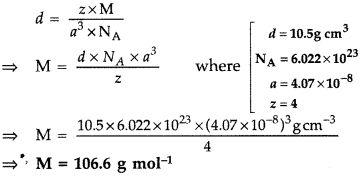Question 99.
(a) Based on the nature of intermolecular forces, classify the following solids: Silicon carbide, Argon
(b) ZnO turns yellow on heating. Why?

(c) What is meant by groups 12-16 compounds? Give an example.
(a) Silicon carbide is a covalent or network solid while Argon is a non-polar molecular solid.
(b) ZnO shows metal excess defect due to presence of extra cations, i.e., Zn2+ ions in interstitial sites which on heating changes into yellow due to loss of oxygen.
ZnO⟶ΔZn2++12O2+2e−
(c) Group 12-16 compounds are imperfect covalent compounds in which the ionic character depends on the electronegativities of the two elements, e.g., ZnS, CdS, etc.

Question 100.
(a) Based on the nature of intermolecular forces, classify the following solids: Benzene, Silver
(b) AgCl shows Frenkel defect while NaCl does not. Give reason.
(c) What type of semiconductor is formed when Ge is doped with Al?

(a) Benzene — Molecular solid (non-polar) Silver — Metallic solid
(b) Due to intermediate radius of AgCl, the size of Ag+ is smaller than larger Na+ ion of NaCl so it can easily occupy interstitial spaces and shows Frenkel defect.
(c) p-type semiconductor is formed when Ge is doped with Al.

Question 101.
(a) Based on the nature of intermolecular forces, classify the following solids: Sodium sulphate, Hydrogen
(b) What happens when CdCl2 is doped with AgCl?
(c) Why do ferrimagnetic substances show better magnetism than antiferromagnetic substances?

(a) Sodium sulphate — Ionic solid
Hydrogen — Molecular solid (non-polar)
(ib) Cd2+ ion is dipositive and therefore addition of one Cd2+ ion results in the loss of two Ag+ ions from the lattice. But out of 2 holes obtained, one is occupied by Cd2+ ion and one left empty. Hence, addition of CdCl2 results in an impurity defect with cation vacancy.
(c) In ferrimagnetism, domains /magnetic moments are aligned in opposite direction in unequal numbers while in antiferromagnetic substances, the domains align in opposite direction in equal numbers so they cancel magnetic moments completely, i.e., net magnetism is zero.

Question 102.
An element crystallises in b.c.c. lattice with cell edge of 400 pm. Calculate its density if 500 g of this element contains 2.5 × 1024 atoms.

Given : a = 400 pm = 400 × 10-10 cm
Z = 2 (for bcc) M = ? d = ?
Using formula, d = Z×Ma3×NA
∵ 2.5 × 1024 atoms of an element have mass = 500 g
∴ 6.022 × 2023atoms of an element have mass= 240.886.4×6.022=240.8838.5408
∴ d = 6.25 g cm-3

Question 103.
An element crystallises in fee lattice with cell edge of 400 pm. Calculate its density if 250 g of this element contain 2.5 × 1024 atoms.

Given : a = 400 pm = 400 × 10-10 cm
Z = 4 (for fee), M = ?, d = ?
Using formula, d = Z×Ma3×NA
∵ 2.5 × 1024 atoms of an element have mass = 250 g
∴ 6.022 × 1023 atoms of an element have mass
= 250×6.022×10232.5×1024
∴ M = 60.22 g
Substituting all values in formula :
d = 4×60.22(400×10−10)3×6.022×1023=240.8838.5408
∴ d = 6.25 g cm-3

Question 104.
An element crystallises in bcc lattice with cell edge of 400 pm. Calculate its density if 250 g of this element contains 2.5 × 1024 atoms.

Given : a = 400 pm = 400 × 10-10 cm
Z = 2 (for bcc), M = ?, d = ?
Using formula, d = Z×Ma3×NA
∵ 2.5 × 1024 atoms of an element have mass = 250g
∴ 6.022 × 1023 atoms of an element have mass
= 250×6.022×10232.5×1024
∴ M = 60.22 g
Substituting all values in formula : .
d = 2×60.22(400×10−10)3×6.022×1023=120.4438.5408
∴ d = 3.125 g cm-3

Question 105.
An element exists in bcc lattice with a cell edge of 288 pm. Calculate its molar mass if its density is 7.2 g/cm3.